# HC Verma Solutions Class 11 Chapter 12 Simple Harmonic Motion

HC Verma Class 11 Solutions Chapter 12 – Simple Harmonic Motion is one of the important chapters for students especially for those appearing in competitive exams like JEE. Besides SHM is an important principle for engineering students. Understanding it is crucial for many factors. In HC Verma book chapter 12, students will deal with this topic and students will come across questions related to finding displacement and acceleration of objects when the amplitude is given. They will further be solving questions on;

• The angular velocity of objects along with finding the position at a certain velocity.
• They will deal with questions on finding the time period of a simple pendulum and maximum force exerted.
• Potential energy experienced by the object when suspended by a string and questions related to the total energy in a simple harmonic motion.

While the book is a very useful study material, students can practice seriously by knowing the right method to solve questions and prepare for competitive exams like JEE Main. The solutions provided here have been crafted by experienced experts and will aid students to understand and develop better problem-solving skills.

Students will mainly learn about topics such as;

1. Simple Harmonic Motion
2. Qualitative Nature of Simple Harmonic Motion
3. Equation of Motion of a Simple Harmonic Motion
4. Terms Associated with Simple Harmonic Motion
5. Simple Harmonic Motion as a Projection of Circular Motion
6. Energy Conservation in Simple Harmonic Motion
7. Angular Simple Harmonic Motion
8. Simple Pendulum
9. Physical Pendulum
10. Torsional Pendulum
11. Composition of Two Simple Harmonic Motions
12. Damped Harmonic Motion
13. Forced Oscillation and Resonance

## Class 11 Important Questions In Chapter 12

1. A particle moving in a circular path with a uniform speed will experience a motion that is (a) simple harmonic (b) oscillatory (c) angular simple harmonic (d)  periodic.

2. Is simple harmonic motion possible in a noninertial frame? Suppose the answer is yes, will the ratio of the force applied with the displacement be constant?

3. Is it possible to achieve negative potential energy in a simple harmonic motion? What will happen in case of zero potential energy at some point other than the mean position?

4. What will happen to the frequency of a spring-mass system when it is taken in an elevator slowly accelerating upward. The only condition given is that the system oscillates with a frequency v.

(a) becomes zero (b) remains the same (c) increases (d) decreases.

5. In one time period, the displacement of a particle in simple harmonic motion will be;

(a) Zero (b) A (c) 2A (d) 4A

## HC Verma Solutions Vol 1 Simple Harmonic Motion Chapter 12

Question 1: A particle executes simple harmonic motion with an amplitude of 10 cm and time period 6 s. At t = 0 it is at position x =5 cm going towards positive x-direction. Write the equation for the displacement x at time t. Find the magnitude of the acceleration of the particle at t = 4 s.

Solution: Amplitude, A = 10cm, r =10cm

Time period, T = 6 sec

W = 2π/T = 2π/6 = π/3 s-1

At t = 0, x = 5 cm

5 = 10 sin(Wx0 + φ) = 10 sin φ

sinφ = 1/2

or φ = π/6

Equation of displacement, x = (10) sin(π/3)

At t = 4 sec

x = 10 sin[π/3 x 4 + π/6] = 10 sin[(8π+π)/6]

= 10 sin(3π/2)

= -10

Acceleration = a = -W2 x = -(π/3) 2 x (-10) = 0.11 cm/s

Question 2: The position, velocity and acceleration of a particle executing simple harmonic motion are found to have magnitudes 2 cm, 1 ms-1 and 10 m s-2 at a certain instant. Find the amplitude and the time period of the motion.

Solution:

x = 2 cm, v = 1 m/s or 100 cm/s, and a = 10 m/s2 or 1000 cm/s2

a = -ω2x

1000 = -2ω2

or ω2 = 500

or ω = 10√5

T = 2π/ω

= 2π/10√5

= 0.28 sec

Now, v2 = ω2 (A2 – x2)

10000 = 5x 100(A2 – 4)

or A = 4.9 cm or -4.9 cm.

Question 3: A particle executes simple harmonic motion with an amplitude of 10 cm. At what distance from the mean position are the kinetic and potential energies equal?

Solution:

Given, K.E. = P.E.

and r = 10 cm

(1/2)mω2(r2-y2) = (1/2)mω2y2

r2 – y2 = y2

=> y = r/√2 = 10/√2 = 5√2 cm form the mean position.

Question 4: The maximum speed and acceleration of a particle executing simple harmonic motion are 10 cm s-1 and 50 cm s-2. Find the position(s) of the particle when the speed is 8 cm s-1.

Solution:

Vmax = rω = 10 cm/s

ω2 = 100/r2 …(1)

And Amax = ω2 r = 50 cm/s

ω2 = 50/y = 50/r …..(2)

From (1) and (2)

100/r2 = 50/r

=> r = 2 cm

Therefore, ω = √100/r2 = 5 s2

Find the positions where the speed is 8m/s

v2 = ω2 (r2 – y2)

=> 64 = 25(4 – y2)

or y = 1.2 cm and -1.2 cm from mean position.

Question 5: A particle having mass 10 g oscillates according to the equation x = (2.0 cm) sin [(100 s-1) t + π/6]. Find (a) the amplitude, the time period and the spring constant (b) the position, the velocity and the acceleration at t = 0.

Solution:

Given equation, x = (2.0 cm) sin [(100 s-1) t + π/6] …(1)

(a) we know, x = A sin(ωt + φ) …(2)

Comparing (1) and (2) we have

A = 2 cm, ω = 100 and φ = π/6

Time period = T = 2π/ω = 2π/10 = π/50 s

For spring constant: ω2 = K/m

or K = ω2 /m = 10/1000 x 1002 = 100 N/m

(b) At t = 0

(1)=> x = 2 sin(π/6)

or x = 1 m

Also v = Aω cos(ωt + φ)

On substituting values, we get

a = 100 m/s2

Question 6: The equation of motion of a particle started at t = 0 is given by x = 5 sin (20 t + π/3), where x is in centimeter and t in second. When does the particle

(a) first come to rest

(b) first have zero acceleration

(c) first have maximum speed?

Solution:

Given equation is: x = 5 sin (20 t + π/3) …(1)

(a) velocity of the particle will be zero at extreme position.

At x = 5

(1)=> 5 = 5 sin (20 t + π/3)

sin (20 t + π/3) = 1 = sin(π/2)

=> 20 t + π/3 = π/2

Or t = π/120 sec

So at π/120 sec it first comes to rest.

(b) a = ω2x = ω2 [5 sin (20 t + π/3)]

For a = 0, 5 sin (20 t + π/3) = 0

=> sin (20 t + π/3) = sin π

=> 20t = π – π/3 = 2 π/3

Or t = π/30 sec

(c) v = A ω cos(ωt+ π/3)

= (20) 5 cos(20t+ π/3)

Here, cos(20t+ π/3) = -1 = cos π [v is maximum]

=> 20t = π – π/3 = 2 π/3

Or t = π/30 sec

Question 7: Consider a particle moving in simple harmonic motion according to the equation

x = 2.0 cos(50 πt + tan-1(0.75))

where x is in centimeter and t in second. The motion is started at t = 0.

(a) When does the particle come to rest for the first time?

(b) When does the acceleration have its maximum magnitude for the first time?

(c) When does the particle come to rest for the second time?

Solution:

x = 2.0 cos(50 πt + tan-1(0.75))

x = 2.0 cos(50 πt + 0.643)

Now, V = dx/dt

= -100 sin(50 πt + 0.643)

As the particle comes to rest for the first time

50 πt + 0.643 = π

Or t = 1.6 x 10-2 sec

(b) Acceleration of the particle = a = dv/dt

=> a = -100π x 50 π cos(50 πt + 0.643)

For maximum acceleration, cos(50 πt + 0.643) = -1 = cosπ

Or t = 1.6 x 10-2 sec

(c) particle comes to rest second time after

(50 πt + 0.643) = 2π

Or t = 3.6 x 10-2 sec

Question 8: Consider a simple harmonic motion of time period T. Calculate the time taken for the displacement to change value from half the amplitude to the amplitude.

Solution:

Using Equation : A = x sin (ωt + φ)

For the first half journey: x = A/2

1/2= sin (ωt + φ)

For φ = 0, ωt = π/6

t = π/6ω

For second journey: x = A/2 to A

x = A sin(ωt + φ)

sin(ωt) = sin (π/2)

or ωt = π/2

or t = π/2ω

Time taken from A/2 to A is

(π/2 )ω – (π/6)ω = (π/3)ω

Now, ω = (2 π/T)

Time taken = π/3(2π/T) = T/6

Question 9: The pendulum of a clock is replaced by a spring-mass system with the spring having spring constant 0.1 Nm-1. What mass should be attached to the spring?

Solution:

T = 2π √(m/k)

T = 2π √(m/0.1)

√0.1 = π √m

or m = 0.01 kg or 10 g

Question 10: A block suspended from a vertical spring is in equilibrium. Show that the extension of the spring equals the length of an equivalent simple pendulum, i.e., a pendulum having frequency same as that of the block.

Solution:

Force due to Gravity = Spring Force.

mg = KA

or K = mg/A

Time Period of the Spring-mass system = T = 2π √(m/k)

= 2π √(mA/mg)

= 2π √(A/g)

Time period for Simple Pendulum = T = 2π √(l/g)

Time period is equal as frequency is equal.

2π √(A/g) = 2π √(l/g)

or A = I (proved)

Question 11: A block of mass 0.5 kg hanging from a vertical spring executes simple harmonic motion of amplitude 0.1 m and time period 0.314 s. Find the maximum force exerted by the spring on the block.

Solution:

x = r = 0.1 m

m = 0.5 kg and T = 0.314 s

Total force exerted on the block = weight of block + spring force

T = 2π √(m/k)

=> 0.314 = 2π √(0.5/k)

Or k = 200 N/m

Force exerted by the spring on the block = F = k x 201.1 x 0.1 = 20 N

Therefore, max force = F + weight = 20 + 5 = 25 N

Question 12: A body of mass 2 kg suspended through a vertical spring executes simple harmonic motion of period 4s. If the oscillations are stopped and the body hangs in equilibrium, find the potential energy stored in the spring.

Solution:

Time period of simple harmonic motion = T = 2π √(m/k)

We are given that, m = 2 kg, T = 4s

=> 4 = 2π √(2/k)

Or k = 5 N/m

We know that, force F is proportional to displacement,

F = mg = kx

=> x = mg/k = 4 m

Thus, P.E. = (1/2) kx2 = 40 J

Question 13: A spring stores 5 J of energy when stretched by 25 cm. It is kept vertical with the lower end fixed. A block fastened to its other end is made to undergo small oscillations. If the block makes 5 oscillations each second, what is the mass of the block?

Solution:

Time period of oscillation = T = 1/v = (1/5) s

P.E. = (1/2)kx2

= (1/2)k(0.25)2

= 5 J

=>k = 160 N/m

Also, T = 2π √(m/k)

Or m = (T/2 π)2 k = 0.16 kg

Question 14: A small block of mass m is kept on a bigger block of mass M which is attached to a vertical spring of spring constant k as shown in the figure. The system oscillates vertically. (a) Find the resultant force on the smaller block when it is displaced through a distance x above its equilibrium position. (b) Find the normal force on the smaller block at this position. When is this force smallest in magnitude? (c) What can be the maximum amplitude with which the two blocks may oscillate together?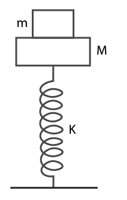Solution:

(a) From free body diagram:

R + mω2x – mg = 0

Resultant force is m ω2x = mg – R

(b) Normal force = R = mg – m ω 2 x

Or ω = √k/(M+m)

Then, R = mg – mkx/(M+m)

m ω 2 x is max for R to be smallest.

X is maximum. The particle should be at the highest point.

(c) Two blocks may oscillate together, R ≥ 0. At limiting R = 0, hen

R = mg – m ω2x = 0

x = mg/m ω = mg(M+m)/mk

Maximum amplitude is, x = g(M+m)/k

Question 15: The block of mass m1 shown in figure (below) is fastened to the spring and the block of mass m2 is placed against it. (a) Find the compression of the spring in the equilibrium position. (b) The blocks are pushed a further distance (2/k) (m1 + m2) g sinθ against the spring and released. Find the position where the two blocks separate. (c) What is the common speed of blocks at the time of separation?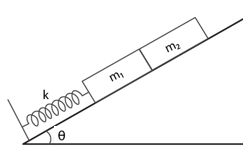Solution: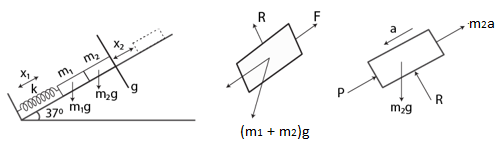(a) At equilibrium condition, kx = (m1+m2)g sin θ

Or x = x1 = [(m1+m2) g sin θ]/k

(b) When the system is released, it will start to make SHM

ω = √[k/(m1+m2)]

When the blocks lose contact, P=0,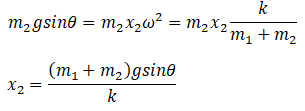blocks will lose contact each other when the springs attain its natural length.

(c) Let v be the common speed attained by both the blocks

(1/2) (m1+m2)v2 = (1/2) (x1+x2)2k – (m1+m2)g sin θ (x1+x2)

Where Total compression = x1+x2

v = [√(k/(m1+m2)) ] g sin θ

Question 16: In figure (below) k = 100 N m-1, M = 1 kg and F = 10 N. (a) Find the compression of the spring in the equilibrium position. (b) A sharp blow by some external agent imparts a speed of 2 m s-1 to the block towards left. Find the sum of the potential energy of the spring and the kinetic energy of the block at this instant. (c) Find the time period of the resulting simple harmonic motion. (d) Find the amplitude. (e) Write the potential energy of the spring when the block is at the left extreme. (f) Write the potential energy of the spring when the block is at the right extreme. The answers of (b), (e) and (f) are different. Explain why this does not violate the principle of conservation of energy.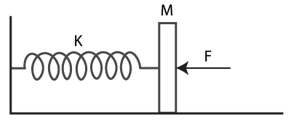Solution:

k = 100 N/m and M = 1 kg and F = 10N

(a) In equilibrium position,

compression, δ = F/k = 10/100 = 0.1 m or 10 cm

(b) The blow imparts speed of 2 m/s to the block towards left.

PE + KE = (1/2) kδ2 + (1/2) Mv2

= (1/2) (100)(0.1)2 + (1/2)(10)(2)2

= 2.5 J

(c) Time period = T = 2π √M/k

= 2π √1/100

= π/5 sec

(d) Let x be the amplitude, which lies between the mean position and the extreme position. So, in the extreme position, compression of the spring is (x + δ)

Since, in the SHM, the total energy remains constant.

(1/2)k(x+ δ)2 = (1/2)k δ2 + (1/2)Mv2 + Fx

= 2.5 + 10x

So, 50(x + 0.1)2 = 2.5 + 10x

Or x = 0.2 m or 20 cm

(e) Potential energy at the left extreme

PE = (1/2)k(x + δ)2 = (1/2) x 100 x (0.2 + 0.1)2 = 4.5 J

(f) Potential energy at the right extreme

P.E. = (1/2)k(x + δ)2 – F(2x)

= 4.5 – 10(0.4)

= 0.5 J

The different values in (b), (e), (f) do not violate law of conservation of energy as the work is done by the external force 10N.

Question 17: Find the time period of the oscillation of mass m in figures 12.E4 a, b, c. What is the equivalent spring constant of the pair of springs in each case?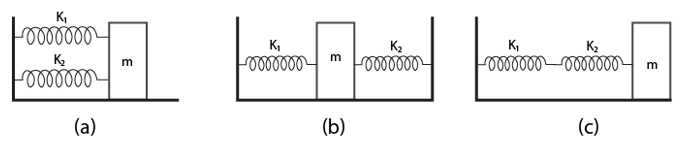Solution:

(a) Equivalent spring constant, k = k1+k2 (parallel)

T = 2π √m/k = 2π √[m/(k1+k2)]

b) When we displace the block m towards left through displacement x,

The resulting force = F = F1 + F2 = (k1+k2)

Now, acceleration = a = F/m = (k1+k2)x/m

The time period can be calculates as: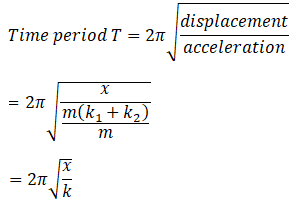c) In series connection equivalent spring constant be k, So

1/k = 1/k1 + 1/k2 = (k1+k2)/ (k1 k2)

Or k = (k1 k2)/ k1+k2)

And time period: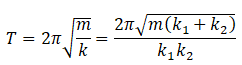Question 18: The spring shown in figure (below) is upstretched when a man starts pulling on the cord. The mass of the block is M. If the man exerts a constant force F, find (a) the amplitude and the time period of the motion of the block, (b) the energy stored in the spring when the block passes through the equilibrium position and (c) the kinetic energy of the block at this position.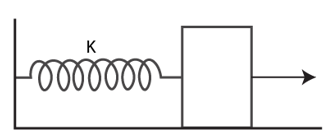Solution:

(a) F = kx

and acceleration = F/m

Time period = T = 2π √m/k

Amplitude =maximum displacement = F/k

(b) The energy stored in the spring when the block passes through the equilibrium position

= (1/2) kx2

= (1/2) k (F/k) 2

= F2/2k

c) At the mean position, potential energy is zero, K.E. = F2/2k

Question 19: A particle of mass m is attached to three springs A, B and C of equal force constant as shown in figure (below). If the particle is pushed slightly against the spring C

and released, find the time period of oscillation.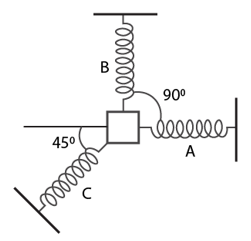Solution:

Consider that particle is pushed slightly against the spring C through displacement x.

Total resultant force on the particle kx due to spring and kx/√2 due to spring A and B, is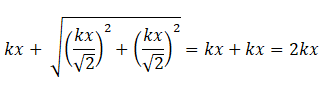Now, acceleration = 2kx/m and Time period = T = 2π √(m/2k)

Question 20: Repeat the previous exercise if the angle between each pair of springs is 120o initially.

Solution:

Total resulting force acting on the mass m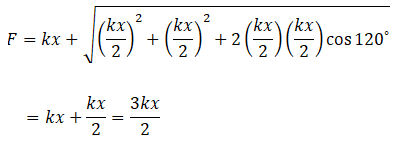Acceleration = a = F/m = 3kx/2m

and a/x = 3k/2

so, ω = √(3k/2m)

Therefore, Time period = T = 2π /ω = 2π x √(2m/3k)

Question 21: The springs shown in the figure (below) are all upstretched in the beginning when a man starts pulling the block. The can exerts a constant force F on the block. Find the amplitude and the frequency of the motion of the block.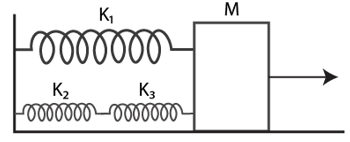Solution:

Since k2 and k3 are in series, let k4 be equivalent spring constant.

So,

1/k4 = 1/k3 + 1/k2 = (k3+k2)/ (k3 k2)

Or k4 = k3k2 /[ k3+k2]

k4 and k1 are parallel. k be equivalent spring constant k.

k = k4 + k1 = k3k2 /[ k3+k2] + k1

= [k3k2 + k1k2 + k3k1]/[ k3+k2]

Now,

T = 2π √(m/k)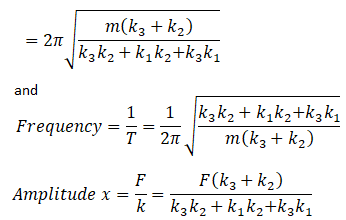Question 22: Find the elastic potential energy stored in each spring shown in figure (below), when the block is in equilibrium. Also find the time period of vertical oscillation of the block.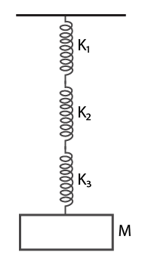Solution:

Since k1 , k2 and k3 are in series, and k be equivalent spring constant.

So,

1/k = 1/k1 + 1/k2 +1/k3

So, k = (k1k2 k3)/ (k3 k2+ k1 k2+ k3 k1)

And , T = 2π /ω = 2π √(m/k)

Substituting the value of k, we have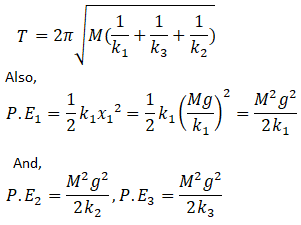[For force = F = Mg,

at spring constant k1, x1 = Mg/K1

Similarly, x2 = Mg/K2 and x3 = Mg/K3 ]

Question 23: The string, the spring and the pulley shown in figure (below) are light. Find the time period of the mass m.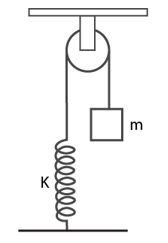Solution:

When mass m is hanging, let l be the extension in the spring.

so, T1 = kl = mg

When a force F is applied, let x be the further extension. This implies

T2 = k(l + x)

Therefore, T = T2 – T1 = k(l + x) – kl = kx

Acceleration = kl/m

and Time period = t = 2π √(m/k)

Question 24: Solve the previous problem if the pulley has a moment of inertia I about its axis and the string does not slip over it.

Solution:

The Initial extension of the spring in the mean position = δ = mg/k

If r is the radius of the pull, then v = rω

At t any instant, total energy for the system executing SHM is constant

(1/2) [mv2 + lω2 + k{(x+δ) 2 2} – mgx] = constant

or (1/2) [mv2 + lω2 + kx2 -kxδ – mgx] = constant

Put the value of v and δ as rω

(1/2) [mv2 + l(v/r) 2 + kx2] = constant

Differentiating above w.r.t. “t” , we get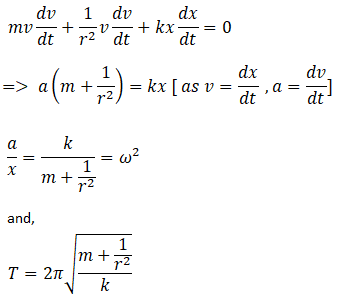Question 25: Consider the situation shown in figure (below). Show that if the blocks are displaced slightly in opposite directions and released, they will execute simple harmonic motion. Calculate the time period.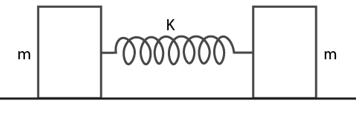Solution:

Here, total energy of the system should be constant.

(1/2)k(2x)2 + (1/2)mv2 +(1/2)mv2 = constant

[The center of mass of the system should not change during the motion. So if the block m on the left side moves towards right side by a distance x, the block on the right side moves towards left side by a distance x. So total compression of the spring is 2x.]

2kx2 + mv2 = constant

derivation of above equation,

(2x)(2k) dx/dt = 2mv dv/dt = 0

or ma + 2kx = 0

or a/x = -2k/m

and ω = √(2k/m)

and T = 2 π √(m/2k)

Question 26: A rectangular plate of sides a and b is suspended from a ceiling by two parallel strings of length L each (figure below). The separation between the strings is d. The plate is displaced slightly in its plane keeping the strings tight. Show that it will execute simple harmonic motion. Find the time period.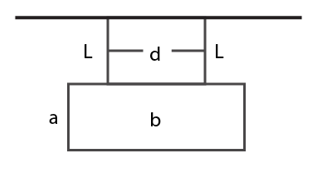Solution:

Driving force = mg sinθ and acceleration = F/m = g sinθ

For small θ, sin θ = 0

Therefore, a = gθ = gx/L

or a is directly proportion to x

So, Motion is simple harmonic, therefore time period: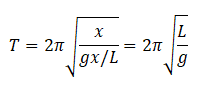Question 27: A 1 kg block is executing simple harmonic motion of amplitude 0.1 m on a smooth horizontal surface under the restoring force of a spring of spring constant 100 N m-1. A block of mass 3 kg is gently placed on it at the instant it passes through the mean position. Assuming that the two blocks move together find the frequency and the amplitude of the motion.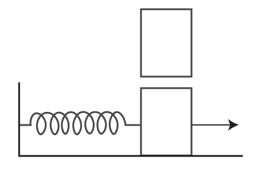Solution:

Here k = 100 N/m

amplitude = 0.1 m and total mass(M) = 1+3 = 4kg

T = 2 π√(M/k) = 2π/5 sec

And, Frequency = v = 5/2π Hz

At the mean position, let v is the velocity of 1 kg block.

K.E. = (1/2) mv2 = (1/2)kx2 = P.E.

or v = 1 m/s

Now, let V be the velocity of the 4 kg weight agin,

v = 4V

or V = (1/4) m/s

Now the two blocks have velocity 1/4 m/s at its mean position.

K.E. = (1/2)MV2 = 1/8 J

When the blocks are going to the extreme position, there will be only potential energy, P.E. = (1/2)kx2 = 1/8

(1/2)(100)x^2 = 1/8

or x = 5 cm

Question 28: The left block in figure (below) moves at a speed u towards the right block placed in equilibrium. All collisions to take place are elastic and the surfaces are frictionless. Show that the motions of the two blocks are periodic. Find the time period of these periodic motions. Neglect the widths of the blocks.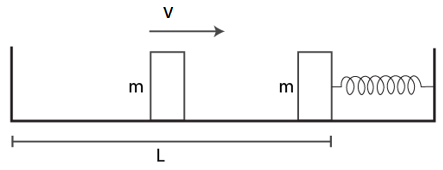Solution: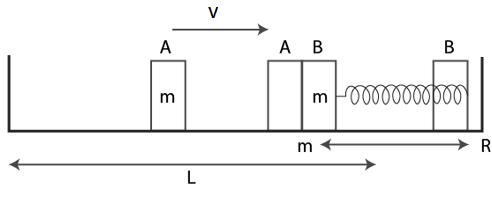As per given statement, collision is elastic and surface is frictionless. When the block A moves with the velocity v and collides with the block B, it transfers all energy to the block B. Block A will move a distance x against a spring, again the block B will return to the original point and completes half of the oscillation.

So, the time period of block B is T i.e.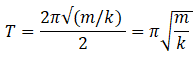Block B collides with block A and comes to rest. Resultantly, block A moves a further distance.

Let us consider “L” be the distance moved by block to return to its original position.

Therefore, time taken by the block to move = L/v + L/v = 2L/v

Time period of the periodic motion is: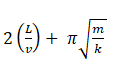Question 29: Find the time period of the motion of the particle shown in figure (below). Neglect the small effect of the bend near the bottom.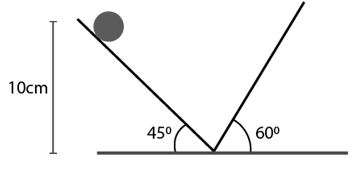Solution: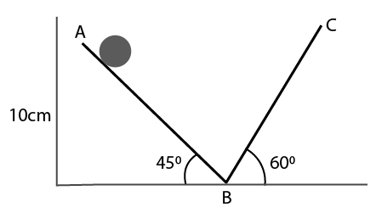Let the time taken to travel AB and BC be t1 and t2 respectively. And, s1 is distance travelled along AB.

Acceleration For part AB = a1 = g sin 45o and s1 = (0.1)/sin45o = 2 m

Let v be the velocity at point B:

Therefore, v2 – u2 = 2 a1 s1

Or v2 = 2 g sin 45o x (0.1)/sin45o = 2

Or v = √2 m/s

Now,

t1 = (v-u)/a1 = 0.2 sec

[on substituting the values]

For Part BC:

A2 = g sin 60o and v = 0 and u = √2 m/s

Also, time taken t2 is: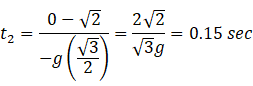Total time period = t1 + t2 = 2(0.2 + 0.15) = 0.7 sec

Question 30: All the surfaces shown in figure (below) are frictionless. The mass of the car is M, that of the block is m and the spring has spring constant k. Initially, the car and the block are at rest and the spring is stretched through a length x when the system is released. (a) Find the amplitudes of the simple harmonic motion of the block and of the car as seen from the road. (b) Find the time period(s) of the two simple harmonic motions.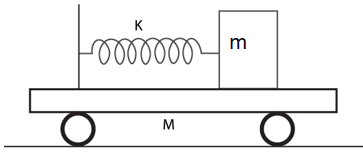Solution:

Let x1, x2 are the amplitude of oscillation of m and M respectively.

(a) mx1 = Mx2

[ law of conservation of momentum]

or (m/M)x1 = x2

And, (1/2) kx02 = (1/2)k(x1 + x2)2

Therefore, xo = x1 + x2

Putting value of x2

xo = x1 + (m/M)x1

=> x1 = Mx0/(M+m)

and x2 = x0 – x1 = mx0/(M+m)

(b)

Let v1, v2 are the velocities of m and M be respectively.

Here v1 of m with respect to M. So, the total energy must be constant.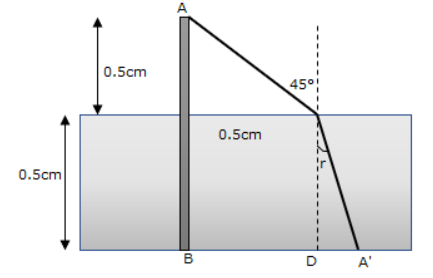………(1)

Again, from law of conservation of momentum,

Mx2 = mx1

=> x1 = (M/m) x2

and Mv2 = m(v1 – v2)

=> v1 – v2 = (M/m)v2

(1)=>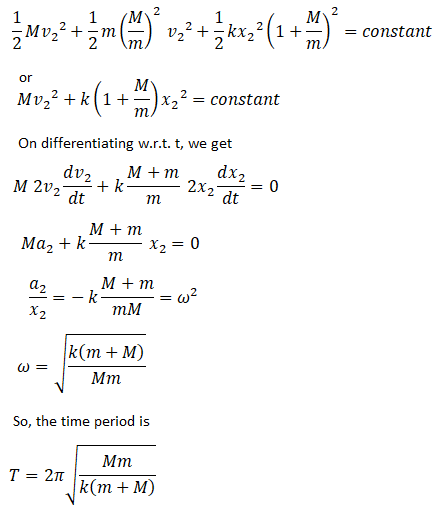Question 31: A uniform plate of mass M stays horizontally and symmetrically on two wheels rotating in opposite directions (figure below). The separation between the wheels is L. The friction coefficient between each wheel and the plate is t. Find the time period of oscillation of the plate if it is slightly displaced along its length and released.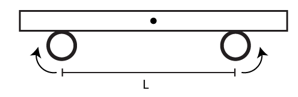Solution:

Let x be the displacement of the uniform plate towards left.

At the displaced position, R1 + R2 = mg

R1(l/2 – x) = R2 (l/2 + x) = (mg – R1) (l/2 + x)

or R1 = [mg(2x+l)]/2l

Now, F1 = μR1 = [μmg(2x+l)]/2l

Similarly, F2 = μR2 = [μmg(2x+l)]/2l

Since F1 > F2

F1 – F2 = ma = [2μmg/l] x

or a/x = 2μg/l = ω2

=> ω = √(μg/l)

Therefore, time period, T = 2π √(l/μg)

Question 32: A pendulum having time period equal to two seconds is called a seconds pendulum. Those used in pendulum clocks are of this type. Find the length of a seconds pendulum at a place where g = π2 m s-2.

Solution:

Given g = π2 m s-2 or 10 m/s and T = 2 s

We know, T = 2π √(l/g)

or l = 1 cm

Question 33: The angle made by the string of a simple pendulum with the vertical depends on time as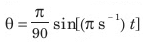Find the length of the pendulum if g = π2 m s-2.

Solution:

On comparing given equation with equation of SHM

ω = π s-1

and T = 2 sec

[As, 2π/T = π]

Again, we know T = 2π√(l/g)

On comparing equations for T

=> l = 1 m, which is the length of pendulum.

Question 34: The pendulum of a certain clock has time period 2.04 s. How fast OF Blow does the clock run during 24 hours?

Solution :

Let time period of given clock = T1 =2.04 sec

We know, time period of normal clock = T = 2.00 sec

Therefore, Change in time lag = T1 – T = 2.04 – 2.00 = 0.04 sec per oscillation

Number of oscillations in a day= N = 24×60×60×0 = 43200

So, total time lag = change in time lag × N

= (0.04) × (43200)

= 1728 sec or 28.8 minutes

Question 35: A pendulum clock giving correct time at a place where g = 9.800 m s-2 is taken to another place where it loses 24 seconds during 24 hours, Find the value of g at this new place.

Solution:

When clock is at g1 it loses 24 seconds in 24 hours

So, T = 2π√(l/g)

=> l = (T2/4π2 ) g …..(1)

This length does not change in the new place T12

l = (T12/4π2 ) g1 …..(1)

From equations (1) and (2)

g1 = (T2/T12) g

= (9.8×4)/(2.0005)2

= 9.746 m/s2

Question 36: A simple pendulum is how many oscillations does it make per second? (b) Whet will be the frequency if the system is taken on the moon where acceleration due to gravitation of the moon is 1.67 m s-2?

Solution:

(a) Here, l = 5m and g = 9.8 m/s2

We know, Time period =T = 2π√(l/g)

= 2(3.14)√(5/9.8) = 4.485

Frequency = f = 1/T = 1/2π x (1.4) = (0.7)/π Hz

(b) Frequency on moon:

fmoon = 1/2π √(l/g) = 1/2π √(l.67/5)

= 1/(2π√3) HZ

Question 37: The maximum tension in the string of an oscillating pendulum m is double of the minimum tension. Find the angular amplitude.

Solution:

Tension in the string is maximum at mean position

Tmax = mg + mv2/l

where m is mass, v = velocity and θ = angular amplitude

Tension is minimum at extreme position = Tmin = mg cosθ

Given: Tmax = 2 Tmin

mg + mv2/l = 2mg cos θ

or cos θ = l/2 + v2/2gl

From the law of conservation energy: v2 = 2gl(1 – cos θ)

Substituting the values, we get

cos θ = (1/2) + [2gl(1-cosθ)]/2gl

or θ = cos-1(3/4)

Question 38: A small block oscillates back and forth on a smooth concave surface of radius R (figure below). Find the time period of small oscillation.Solution:

Let “m” be the mass of the block and “R” Radius of the concave surface.

Also, Driving force F = mgsinθ

Therefore, a = g sinθ

[for small angle, sinθ = θ]

So, a = gθ = 1(approx) …(1)

and

If x is the displacement of block from mean postion then sinθ = x/R

θ = x/R (approx)

(1)=> a = gx/R ….(2)

From equation of SHM, a = ω2x ….(3)

From (2) and (3), ω = √g/R

Time period=T = 2π/ω = 2π (√R/g)

Question 39: A spherical ball of mass m and radius r rolls without slipping on a rough concave surface of large radius R. It makes small oscillations about the lowest point. Find the time period.

Solution: Torque on the ball = F x perpendicular distance

= (mg sinθ) r

Moment of inertia of ball = I = I_cm + mr2

For spherical surface: Icm = (2/5) mr2

Therefore, I = (2/5) mr2 + mr2

= (7/5) mr2

Angular acceleration of a ball = α = [Torque on the ball]/I

= 5gsinθ/7r

Now, Angular acceleration about the center of the surface:

At effective distance, R-r : αo = 5gsinθ/7(R-r)

For small angle, sinθ=θ(approx)

Again, linear acceleration, a = αr = 5gsinθ/7r . r

= 5gsinθ/7

Question 40: A simple pendulum of length 40 cm is taken inside a deep mine. Assume for the time being that the mine is 1600 km deep. Calculate the time period of the pendulum there. Radius of the earth = 6400 km.

Solution:

Let M be the mass of earth: M = ρ vg = GM/R2 = (4/3)πR3 ρ ….(1)

Gravity at depth ‘d’ :

g’ = GM’/r2

Where r is the distance from center of the earth to that point.

r = R – 1600 = 6400 – 1600 = 4800 km

and M’ = (4/3)πr3 ρ …..(2)

From (1) and (2)

M/M’ = R3/r3

or M’ = (Mr3)/R3

Therefore, g’ = G/r2 . (Mr2/R3) = GM/R2 . r/R

g’ = g.r/R

= [9.8x4800x10^3]/[6400×10^3]

= 7.35 m/s2

Time period = T = 2π√(l/g’)

= 2π √(0.4/7.35)

= 1.47 s

Question 41: Assume that a tunnel is dug across the earth (radius =R) passing through its centre. Find the time a particle takes to cover the length of the tunnel if (a) it is projected into the tunnel with a speed of √gR (b) it is released from a height R above the tunnel (c) it is

thrown vertically upward along the length of tunnel with a speed of √gR.

Solution:

(a) Time period of the oscillation of a particle and center of the earth acts as mean position.

Time period = T = 2π/ω = 2π √(R/S)

Velocity = v = ω √(A2-R2)

Where “A” be the amplitude.

√SR = √(S/R) √(A2-R2)

Solving above, we have

or A = √2 R

Let t1 time when particle is at distance ‘R’

Form equation, y = A sin ωt

R = √2 R sin ωt1

or ωt1 = 3π/4

Let t1 time when particle is at distance ‘-R’

-R = √2 R sin ωt2

or ωt2 = 5π/4

So, ωt2 – ωt1 = π/2

or t2 – t1 = π/2ω = π/2 . √R/g

(b)

When body is dropped from a height ‘R’. Final velocity of the body on reaching the ground is ‘v’ is:

(1/2) mv2 = GMm/(R+R)

[change in kinetic energy = change in potential energy]

v2 = gR

or v = √gR

Therefore, time taken to cover the length of tunnel will be same,[as in part (a)]

t2 – t1 = π/2 . √R/g

(c)

It projected upward and reaches back to the surface with the same velocity i.e. √gR

From there it enters tunnel with same velocity as in part (a) and hence time taken to cover the tunnel.

Question 42: Assume that a tunnel is dug along a chord of the earth, at a perpendicular distance R/2 from the earth’s centre where R is the radius of the earth. The wall of the tunnel is frictionless. (a) Find the gravitational force exerted by the earth on a particle of mass m placed in the tunnel at a distance x from the centre of the tunnel. (b) Find the component of this force along the tunnel and perpendicular to the tunnel. (c) Find the normal force exerted by the wall on the particle. (d) Find the resultant force on the particle. (e) Show that the motion of the particle in the tunnel is simple harmonic and find the time period.

Solution:

(a)

Mass of the earth with reduced radius ‘l’:

M’ = (4/3) πl3ρ

where ‘l’ be the distance from the center of the earth to the particle at distance “x”

Mass of the earth with radius R

M = (4/3)πR3 ρ

Now, M’/M = l3/R3

or M’ = M(l/r) 3

The gravitational force on the particle will be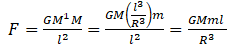…(1)

Also,

l2 = x2 + (R2/2) 2

l = (x2 + R2/4)(1/2)

(1)=>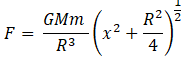(b) Let the component of force along tunnel:

Fx = F cos θ = Fx/l

= GMm/R3 . x

Let the perpendicular component be Fy

Fy = F sin θ = FR/2l

= GMm/2R2 . x

(c) the walls exert the same amount of force which the particle exerts on them

FN = FY

= GMm/2R2

(d) Resultant force on particle is the same force that is acting along the tunnel

Fr = Fx = GMm/R3 . x

(e) For a body to be in S.H.M, a directly proportional to x

a = Fx/m = GMm/MR3 . x

= GM/R3 . x

If GM/R3 is constant

a = kx

=> a α x

Question 43: A simple pendulum of length is suspended through the ceiling of an elevator. Find the time period of small oscillations if the elevator (a) is going up with an acceleration a0 (b) is going down with an acceleration a0 and (c) is moving with a uniform velocity.

Solution:

(a) Time period = T = 2π √(l/g)

Replace g value by net acceleration.

l/g = 4/π2

or l = 4/π2 . g ….(1)

When car accelerates with acceleration a_o, net acceleration on the bob:

a = √(g2 + ao2)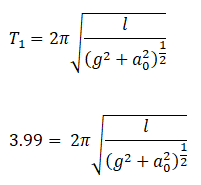l = (3.99) 2/4π2 [g2 + ao2]1/2 ….(2)

From (1) and (2)

4/π2 . g = (3.99) 2/4π2 [g2 + ao2] 1/2

or ao = 0.1 g (approx)

Question 44: simple pendulum of length 1 feet suspended from the ceiling of an elevator takes π/3 seconds to complete one oscillation. Find the acceleration of the elevator

Solution:

If a0 be the elevator is moving with acceleration.

Time period = T = 2π√[l/(g+a0)]

π/3 = 2π√[l/(g+a0)]

1/6 = √[l/(g+a0)]

g + a0 = 36 l

[Here g = 32 ft/s2]

ao = 4 ft/s2

Question 45: A simple pendulum fixed in a car has a time period of 4 seconds when the car is moving uniformly on a horizontal road. When the accelerator is eased, the time period changes to 3.99 seconds. Making an approximate analysis, find the acceleration of the car.

Solution:

When car is moving with uniform velocity:

T = 2π √(l/g)

l = 4/π2 . g . ….(1)

The net acceleration on the bob, when car accelerates with acceleration ao

a = √(g2+ao2)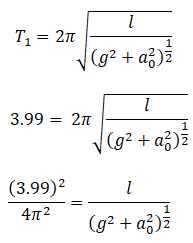l = (3.99)2/4π2 (g2 + ao2)1/2 ….(2)

From (1) and (2)

4/π2 . g = (3.99) 2/4π2 (g2 + ao2)1/2

ao = 0.1 g (approx)

Question 46: A simple pendulum of length i is suspended from the ceiling of a car moving with a speed u on a circular horizontal road of radius r. (a) Find the tension in the string when it is at rest with respect to the car. (b) Find the time period of small oscillation.

Solution:

The tension in the string will be due to force due to gravity and Force due to centripetal force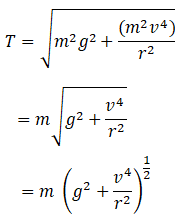a = (g2 + v4/r2)1/2 is the acceleration on the bob.

(b) Time period of the small oscillation

T = 2π √(l/a)

a = (g2 + v4/r2)1/2

and Time period,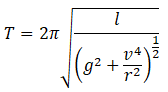Question 47: The ear-ring of a lady shown in figure (below) has a 3 cm long light suspension wire (a) Find the time period of small oscillations if the lady is standing on the ground. (b) The lady now sits in a merry-go-round moving at 4 m s-1 in a circle of radius 2 m. Find the time period of small oscillations of the ear-ring.

Solution:

(a) Length = l = 3cm or 0.03m and g = 9.8

Time period = T = 2π √(l/g)

= 0.3476 sec

A centripetal force also acts on the earring = Fc = mv2/r and v = 4m/s

The resultant acceleration on the ear-ring:

a = √(g2+v4/r2)

Therefore, Time period = T = 2π√(l/a)

= 0.30 sec

Question 48: Find the time period of small oscillations of the following systems. (a) A metre stick suspended through the 20 cm mark. (b) A ring of mass m and radius r suspended through a point on its periphery. (e) A uniform square plate of edge a suspended through a corner. (d) A uniform disc of mass m and radius r suspended through a point r/2 away from the centre.

Solution:

(a) Time period for a physical pendulum = T = 2π √(I/mgl) …(1)

let I is the distance between centre of mass and point of suspension

I = Icm + mx2 = 0.5 – 0.2 = 0.3 m

Now, I = mi2/12 + m(0.3) 2

= 0.08 + 0.09

= 0. 17 m

Therefore, T = 1.51 sec

(on putting values in (1))

(b) when a ring is suspended through a point on its periphery,

I = Icm + mr2

= mr2 + mr2

I = 2 mr2 …(2)

[here , I_cm of circle = mr2]

Now, If l = r,

T = 2π √(I/mgr)

using (2),

T = 2π √(2r/g)

(c) when axis pass through corner of the square

Iz = Ix + Iy + mr2

= ma2/12 + ma2/12 + ma2/12

= 2ma2/3

Now,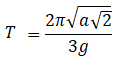(d) Uniform disc of a mass m and radius r suspended through a point r/2 away from cente.

Here l = r/2

I = Icm + ml2

= mr2/2 + m(r/2) 2

= (3/4) mr2

Now,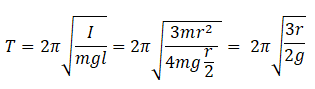Question 49: A uniform rod of length l is suspended by an end and is made to undergo small oscillations. Find the length of the simple pendulum having the time period equal to that of the rod.

Solution:

Time period for a physical pendulum = T = 2π √(I/mgr) …(1)

Where, r = distance between center of mass and point of suspension

Given, r = l/2

I = I_cm + ml2

= ml2/12 + ml2/4

= ml2/3

Therefore,

(1)=> T = 2π √(2l/3g) …(2)

Again, time period of simple pendulum = T = 2π √(L/g) ….(3)

From (1) and (2)

L = 2l/3

Question 50: A uniform disc of radius r is to be suspended through a small hole made in the disc. Find the minimum possible time period of the disc for small oscillations. What should be the distance of the hole from the centre for it to have minimum time period?

Solution:

Let x be the distance between centre of mass and point of suspension, choose any.

I = Icm + mx2

= mr2+ mx2

Now, time period is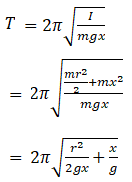The condition for minimum of T : dt/dx = 0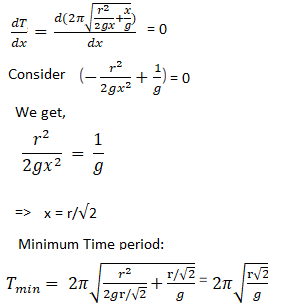Question 51: A hollow sphere of radius 2 cm is attached to an 18 cm long thread to make a pendulum. Find the time period of oscillation of this pendulum. How does it differ from the time period calculated using the formula for a simple pendulum?

Solution:

Length of the pendulum = l = 18 + 2 = 20 cm

and, r = 0.02

Time period : T = 2π √(I/mgl)

Where l is the distance between center of mass and point of suspension.

I = Icm + ml2

= (2/3) mr2 + ml2

= 0.0403 m

and

T = 2π √[(0.0403/(0.20mg)]

= 0.9009 sec

If we consider simple pendulum equation, then time period is

T1 = 2π √l/g

= 2π √(0.2/9.8

= 0.897 sec

Difference in time: T – T1

= 0.9009 – 0.897

= 0.0033 sec

Percentage of Time = 0.3 % more than simple pendulum.

Question 52: A closed circular wire hung on a nail in a wall undergoes small oscillations of amplitude 2° and time period 2 s. Find (a) the radius of the circular wire, (b) the speed of the particle farthest away from the point of suspension as it goes through its mean position, (c) the acceleration of this particle as it goes through its mean position and (d) the acceleration of this particle when it is at an extreme position. Take g = π2 m s-1.

Solution:

(a) Time period = T = 2π √(I/mgl) …(1)

I = Icm + mr2

= mr2 + mr2

= 2 mr2

At T = 2 sec

(1)=>

r = 50 cm (approx)

(b) From law of conservation of energy

Δ KE = Δ PE

or (1/2) Iω2 – 0 = mgr(1-cosθ)

At θ = 2o

ω2 = 0.11 m/s

(c) Acceleration of the particle is due to centripetal force on the circle.

a = v2/2r

here v = 0.11 and r = 0.5

=> a = 0.012 m/s2

(d) Acceleration of particle when it is at extreme position,

Gicen: g = π2 m s-1 and T = 2 sec

Here T = 2π/ω

Angular acceleration = α = ω2 θ

= (π2 x 2π)/180

= π3/90

Linear acceleration = a = α(2r) = 34 cm/s2

[here r = 100]

Question 53: A uniform disc of mass m and radius r is suspended through a wire attached to its centre. If the time period of the torsional oscillations be T, what is the torsional constant of the wire?

Solution:

Moment of inertia about centre of mass of a uniform disc

I = mr2/2

Now, Time period T = 2π √(I/k); where K torsional constant

Therefore, K = 4π2I/T2

or K = 2π2mr2/T2

Question 54: Two small bails, each of mass m are connected by a light rigid rod of length L. The system is suspended from its centre by a thin wire of torsional constant k. The rod is rotated about the wire through an angle θ0 and released. Find the force exerted by the rod on one of the balls as the system passes through the mean position.

Solution:

Torque on the rod (τ) is directly proportion to θ

Therefore, τ = Kθ where k is torsional constant

Now, the work done, W is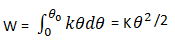From work energy theorem,

(1/2) Iω2 = K θ2/2 where I = mL2/2

= √(2Kθ2)/(mL2)

Tension in the string is due to centripetal force and weight and resultant is,

T = √(F2m2g2)

= √[(mrω2) 2 + m2g2]

or T = √[(K2θ4/L2) + m2g2]

Question 55: A particle is subjected to two simple harmonic motions of same time period in the same direction. The amplitude of the first motion is 3.0 cm and that of the second is 4.0 cm. Find the resultant amplitude if the phase difference between the motions is (a) 0°, (b) 60o, (c) 90o.

Solution:

(a) amplitudes of S.H.M

Both S.H.Ms have same time period and direction and hence their equations

y1 = A1 sin ωt and y2 = A2 sin ωt

Resultant S.H.M equation, y = y1 + y2

=> y = 3 sin ωt + 4 sin ωt = 7 sin ωt

Amplitude of resultant S.H.M is 7cm

(b) S.H.Ms have a phase difference of π/3 between them

y1 = A1 sin ωt and y2 = A2 sin ωt + π/3

Resultant amplitude = A2 = A12 + A22 + 2A1 A2 cos(π/3)

A2 = 9 + 16+2x3x4x(1/2)

or A = 6.1 cm

(c) At phase difference π/2

y1 = A1 sin ωt and y2 = A2 sin ωt + π/2

Resultant amplitude = A2 = A12 + A22 + 2A1 A2 cos(π/2)

A2 = 9 + 16+2x3x4x0

or A = 5 cm

Question 56: Three simple harmonic motions of equal amplitudes A and equal time periods in the same direction combine. The phase of the second motion is 60o ahead of the first and the phase of the third motion is 60° ahead of the second. Find the amplitude of the resultant motion.

Solution:

S.H.Ms are

x1 = A sin ωt and x2 = A sin(ωt+π/3) and x3 = A sin(ωt+2π/3)

Resultant, x = x1 + x2 + x3

Resultant amplitude between first two => R = x1 + x2

R = √3 A

Resultant amplitude of all three S.H.Ms will be the resultant of R and x3

Let R’ be the resultant of all three S.H.Ms

R’ = √[A32 + R2 + 2A3 R cos(π/2)]

= 2A

Question 57: A particle is subjected to two simple harmonic motions given by x1 = 2.0 sin(100 πt) and x2 = 2.0 sin(120 πt + π/3), where x is in centimeter and t in second. Find the displacement of the particle at (a) t = 0.0125, (b) t = 0025.

Solution:

Given:

x1 = 2.0 sin(100 πt) and x2 = 2.0 sin(120 πt + π/3), where x is in centimeter and t in second.

Resultant wave = x = x1 + x2

x = 2[sin 100πt + sin (120πt + π/3)]

(a) At t = 0.0125 sec

x = 2[sin 100π(0.0125) + sin (120π(0.0125) + π/3)]

= – 2.41 cm

(b) At t = 0.025 sec

x = 2[sin 100π(0.025) + sin (120π(0.025) + π/3)]

= 0.27 cm

Question 58: A particle is subjected to two simple harmonic motions, one along the X-axis and the other on a line making an angle of 45^o with the X-axis. The two motions are given by

x = x0 sin ωt and s = s0 sin ωt

Find the amplitude of the resultant motion.

Solution:

x = x0 sin ωt and s = s0 sin ωt and angle = 45o

Amplitude of resultant motion = R2 = x2 + s2

= x02 + s02 + 2x0 s0 cos(45o)

or R = [x02 + s02 + √2 x0 s0 ](1/2)